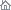Home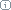About Us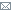ContactBlogWhat's NewProductsBuy NowDownloadsForumGeneXproTools Online Guide Learn how to use the 5 modeling platforms of GeneXproTools with the Online GuideLast update: February 19, 2014Missing Values Mapping

GeneXproTools supports missing values both for numerical and categorical variables. The supported representations for missing values consist of NULL, Null, null, NA, na, ?, blank cells, ., ._, and .*, where * can be any letter in lower or upper case.

When data is loaded into GeneXproTools, the missing values are automatically replaced by zero so that you can start modeling right away. But then GeneXproTools allows you to choose different mappings through the Missing Values Mapping Window.

In the Missing Values Mapping Window you have access to pre-computed data statistics, such as the majority class for categorical variables and the average for numerical variables, to help you choose the most effective mapping.

As mentioned for categorical values, GeneXproTools is not just a useful platform for trying out different mappings for missing values to see how they impact on model evolution and then choose the best one: GeneXproTools generates code with support for missing values that you can immediately deploy without further hassle, allowing you to use the exact same format that was used to load the data into GeneXproTools. The sample MATLAB code below shows a classification model of 7 variables, 6 of which with missing values:
```
%------------------------------------------------------------------
% Classification model generated by GeneXproTools 5.0 on 5/17/2013 6:44:02 PM
% GEP File: D:\GeneXproTools\Version5.0\OnlineGuide\Diabetes_M01.gep
% Training Records:  570
% Validation Records:   198
% Fitness Function:  ROC Measure, ROC Threshold
% Training Fitness:  801.044268510405
% Training Accuracy: 75.09% (428)
% Validation Fitness:   842.459561470235
% Validation Accuracy:  77.27% (153)
%------------------------------------------------------------------

function result = gepModel(d_string)

ROUNDING_THRESHOLD = 1444302.57350085;

G1C9 = 8.49354625080111;
G2C0 = -3.496505630665;
G2C6 = 0.893559068575091;
G3C6 = 4.40351573229164;

d = TransformCategoricalInputs(d_string);

varTemp = 0.0;

varTemp = ((gep3Rt(((d(4)-d(1))^3))-(d(8)*(d(6)-G1C9)))^2);
varTemp = varTemp + (((((G2C0+d(2))/2.0)*(d(2)+d(2)))+((G2C6+d(5))*G2C0))*d(2));
varTemp = varTemp + (gep3Rt((d(2)*(((G3C6-d(2))*d(3))-d(5))))^3);

if (varTemp >= ROUNDING_THRESHOLD),
result = 1;
else
result = 0;
end

function result = gep3Rt(x)
if (x < 0.0),
result = -((-x)^(1.0/3.0));
else
result = x^(1.0/3.0);
end

function output = TransformCategoricalInputs(input)
switch char(input(1))
case '.D'
output(1) = 12.0;
case '.E'
output(1) = 11.0;
case '.L'
output(1) = 15.0;
case '.T'
output(1) = 10.0;
case '.Z'
output(1) = 0.0;
otherwise
output(1) = str2double(input(1));
end

switch char(input(2))
case '?'
output(2) = 0.0;
otherwise
output(2) = str2double(input(2));
end

switch char(input(3))
case '?'
output(3) = 0.0;
otherwise
output(3) = str2double(input(3));
end

switch char(input(4))
case '?'
output(4) = 0.0;
otherwise
output(4) = str2double(input(4));
end

switch char(input(5))
case '?'
output(5) = 0.0;
otherwise
output(5) = str2double(input(5));
end

switch char(input(6))
case '?'
output(6) = 0.0;
otherwise
output(6) = str2double(input(6));
end

output(8) = str2double(input(8));
```

Related Tutorials:

Related Videos:

 Leave Feedback Please enter the number below using the combo boxes before sending your feedback. 3 8 4 0 1 2 3 4 5 6 7 8 9   0 1 2 3 4 5 6 7 8 9   0 1 2 3 4 5 6 7 8 9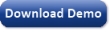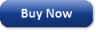Time Limited Trial

Released February 19, 2014

Last update: 5.0.3883New Entries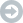Subscribe to the GEP-List

 3 8 4 0 1 2 3 4 5 6 7 8 9   0 1 2 3 4 5 6 7 8 9   0 1 2 3 4 5 6 7 8 9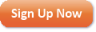Home | What's New | Products | Buy Now | Downloads | Quick Tour | Support | Contact Us | About Gepsoft | Sign UpForum | Blog | Videos | Tutorials | Server Knowledge Base | Logistic Regression Guide | Terms of Use | Privacy & Cookies